# Contravariant tensor

of valency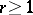A tensor of type, i.e. an element of the tensor productofcopies of a vector spaceover a field. The space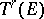is a vector space overwith respect to the operations of addition of contravariant tensors of the same valency and multiplication of them by a scalar. Letbe a finite-dimensional vector space with basis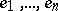. Then the dimension ofis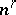; one possible basis inis given by all possible contravariant tensors of the form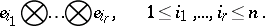Any contravariant tensor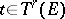can be represented in the formThe numbersare called the coordinates or components ofwith respect toin. On changing to a new basis inaccording to the formulasthe components ofchange according to the so-called contravariant lawWhen the valencyequals 1, a contravariant tensor is the same as a vector, that is, an element of; when, a contravariant tensor can be related in an invariant way with an-linear mapping intoof the direct product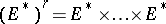ofcopies of the dual space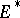to. For this it suffices to take as the components of the contravariant tensorthe values of the-linear mappingat(where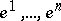are the basis elements indual to, that is,), and conversely. For this reason contravariant tensors are sometimes directly defined as multilinear functionals on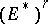.

More generally, letbe a commutative ring with unit element anda unitary module over. Then the elements of the-fold tensor product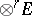are called-contravariant tensors or contravariant tensors of valency or order. The phrase "contravariant tensor of order r" is also used to denote a contravariant tensor field of orderover a smooth manifold; cf. Tensor bundle. Such a field assigns to each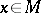an element of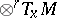, the-fold tensor product of the tangent space toat. In the setting of rings and modules such a tensor field is simply an-contravariant tensor of the moduleof sections of(i.e. the vector fields) over the ring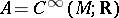of smooth functions on.